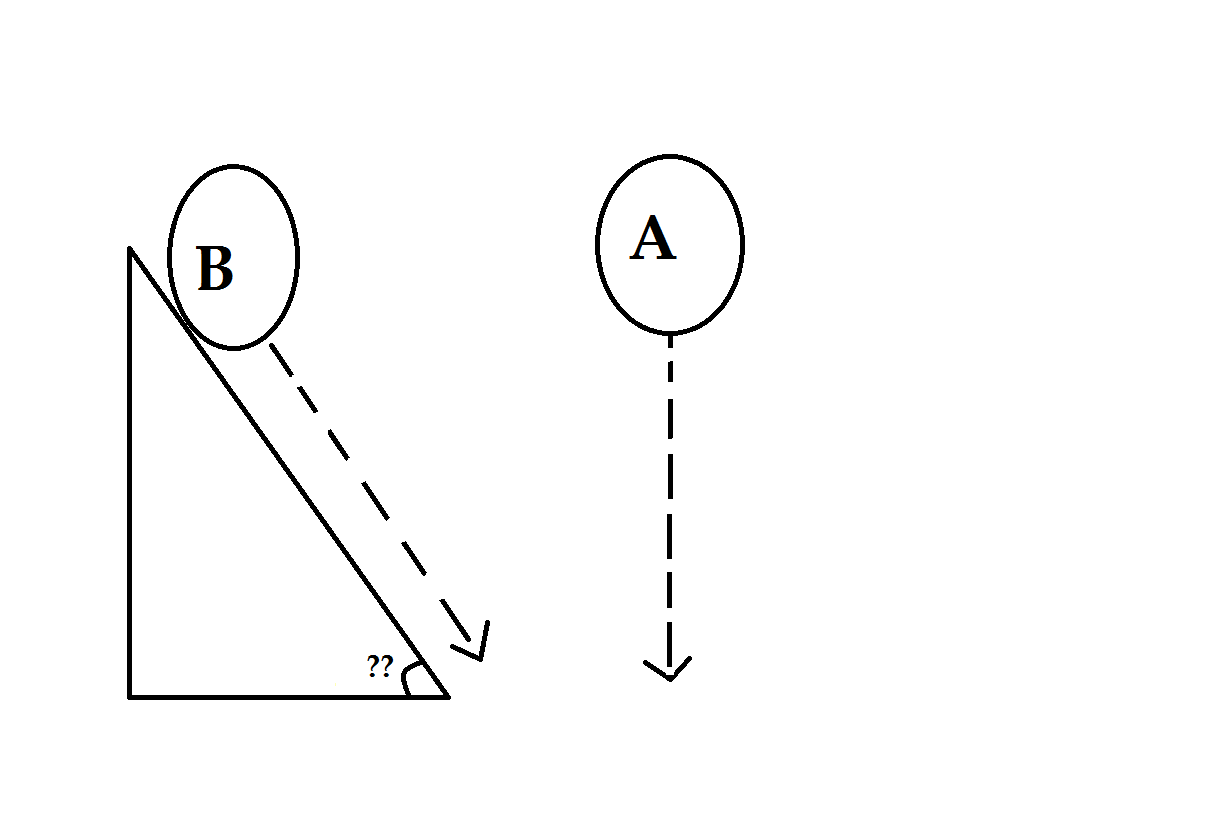# Newton's Logical MechanicsTwo balls $A$, and $B$ are kept at a certain height from where ball $A$ is dropped freely and Ball $B$ is rolled through a wedge with coefficient of friction $\mu=\dfrac{21}{32}$ . Given that $v_{A}$, $v_{B}$ are the final velocities of balls $A$, and $B$ respectively with a relation that. $v_{A} =2\sqrt{2}v_{B}$ Find the angle of inclination of the wedge in degrees, rounded to the nearest integer

Note:

• The balls are identical

• Ball B is not Rolling

×

Problem Loading...

Note Loading...

Set Loading...